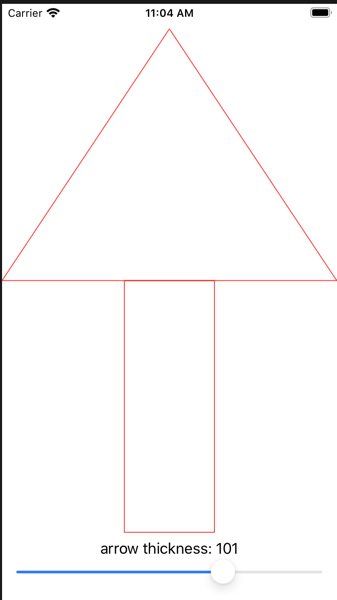# Day 43, 44, 45, 46 of #100DaysOfSwiftUIStart 100DaysOfSwiftUI from 2020.Mar.18th.

Day 43, 44, 45, 46: Project 9, part one/two/three/four
done with 2.5 hours

New findings: followings are new findings

• I wonder why path(in rect: CGRect) will receive rect from frame modifier, but it would come from Shape, I guess.
• ImagePaint, I'm not sure when I'll use it, but looks nice for excellent UI
• animationData works without adding further code. super!

Here is the code except Challenge 3.
# I could not have much interest in ColorCycling...struct ContentView: View {
@State private var thickness : CGFloat = 50.0

var body: some View {
VStack(spacing: 0) {
Spacer()

Arrow(thickness: thickness)
.stroke(Color.red, lineWidth: 1)
.animation(.default)

Spacer()

Group {
Text("arrow thickness: \(Int(thickness))")
Slider(value: \$thickness, in: 1...150, step: 5)
}
}
}
}

struct Arrow: Shape {
var thickness: CGFloat
var animatableData: CGFloat {
get { thickness }
set { self.thickness = newValue }
}

func path(in rect: CGRect) -> Path {
var path = Path()

// triangle
path.move(to: CGPoint(x: rect.midX, y: rect.minY))
path.addLine(to: CGPoint(x: rect.minX, y: rect.maxY/2))
path.addLine(to: CGPoint(x: rect.maxX, y: rect.maxY/2))
path.addLine(to: CGPoint(x: rect.midX, y: rect.minY))

let rectXstart = rect.midX - thickness / 2
let rectXend   = rect.midX + thickness / 2

// rectangle
path.move(to: CGPoint(x: rectXstart, y: rect.maxY/2))
path.addLine(to: CGPoint(x: rectXstart, y: rect.maxY))
path.addLine(to: CGPoint(x: rectXend, y: rect.maxY))
path.addLine(to: CGPoint(x: rectXend, y: rect.maxY/2))
path.addLine(to: CGPoint(x: rectXstart, y: rect.maxY/2))
return path
}
}